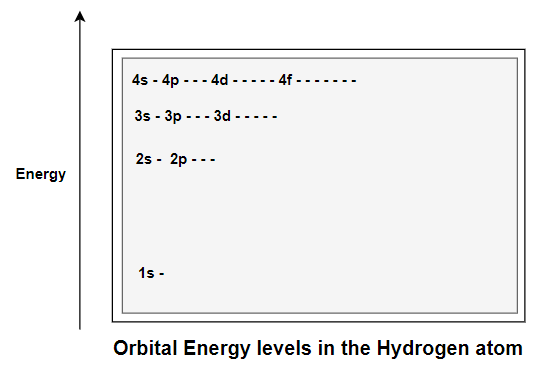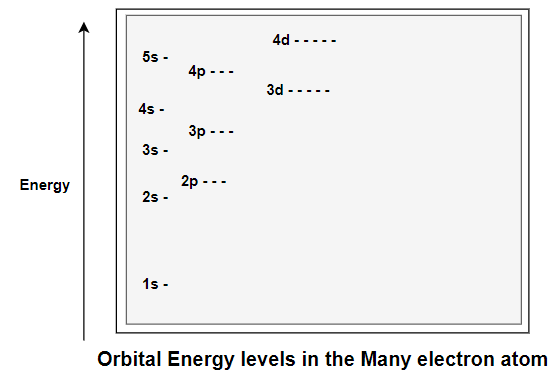# Energy of Orbitals:

## Energy of Orbitals

The energy which is essential to take an electron present in that orbital to infinity or the release of energy when an electron from an infinity it is added to that orbital, it is referred to as the energy of orbitals.

Energy of the electrons is different in Hydrogen Atom (Single Electron Atom) and Multi-electron atom.Lets take a look at each of them

### Energy of Orbital in Hydrogen atom

• The energy of an electron in a hydrogen atom is determined solely by the principal quantum (n). Thus the energy of the orbitals in hydrogen atom increases as follows :
1s < 2s = 2p < 3s = 3p = 3d <4s = 4p = 4d = 4f <..• Although the shapes of 2s and 2p orbitals are different, an electron has the same energy when it is in the 2s orbital as when it is present in 2p orbital. The orbitals having the same energy are called degenerate orbitals
What are Degenerate orbitals Orbitals having the same energy are called degenerate orbitals.
• The 1s orbital in a hydrogen atom is the most stable condition and is called the ground state and an electron residing in this orbital is most strongly held by the nucleus. An electron in the 2s, 2p or higher orbitals in a hydrogen atom is in excited state

### Energy of Orbital in multi-electron atoms

• The energy of the orbital in multi-electron atoms depends on angular momemtum quantum number as well as Principle quantum number
• For a given principal quantum number, s, p, d, f ... all have different energies. Within a given principal quantum number, the energy of orbitals increases in the order s The reason behind different energies between the various subshells of the same shell is that there exists a mutual repulsion among the electrons in multi-electron atoms. The stability of multi-electron atom is due to the bigger magnitude of attractive force between nucleus and electrons as compared to the forces of repulsion between electrons of the inner shell and outer shell.This is known as the shielding of the outer shell electrons from the nucleus by the inner shell electrons
Shielding effect or screening effect Due to the presence of electrons in the inner shells, the electron in the outer shell will not experience the full positive charge on the nucleus.
So, due to the screening effect, the net positive charge experienced by the electron from the nucleus is lowered and is known as effective nuclear charge. Effective nuclear charge experienced by the orbital decreases with increase of azimuthal quantum number (l).
• The energy of the orbital is determined by sum of the principle quantum number and Azimuthal quantum number. (n + l)
• Here is the orbital energy levels in many-electron atom as per above pointsQuestion 1
The orbital with maximum energy is
(a) 3d
(b) 5p
(c) 4s
(d) 6d
Solution
(d) as per n+l rule

Question 2
Energy of atomic orbitals in a particular shell is in the order
(a) s (b) s>p>d>f
(c) p (d) f>d>s>p
Solution
(a) as per n+l rule

Question 3
The energy of an electron of 2py orbital is
(a) Greater than that of $2p_x$ orbital
(b) Less than that of $2p_x$ orbital
(c) Equal to that of 2s orbital
(d) Same as that of $2p_z$ orbital
Solution
(d)